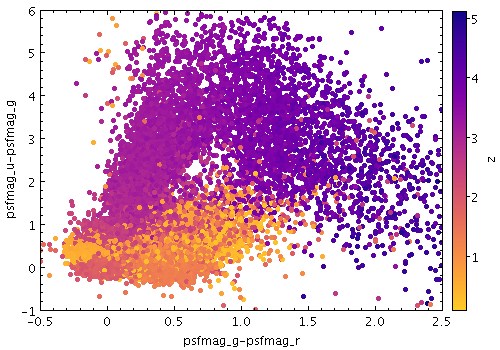Next Previous Up Contents
Next: weighted
Previous: density

#### 8.4.6 `aux`

Paints markers in a colour determined by the value of an additional data coordinate. The marker colours then represent an additional dimension of the plot. You can also adjust the transparency of the colours used. The way that data values are mapped to colours is usually controlled by options at the level of the plot itself, rather than by per-layer configuration.

Usage:

```   shadingN=aux auxN=<num-expr> auxnullcolorN=<rrggbb>|red|blue|...
opaqueN=<number>
```

All the parameters listed here affect only the relevant layer, identified by the suffix `N`.

Example:```   stilts plot2plane layer1=mark in1=dr5qso.fits
x1=psfmag_g-psfmag_r y1=psfmag_u-psfmag_g size1=2
xmin=-0.5 xmax=2.5 ymin=-1 ymax=6```

Associated parameters are as follows:

`auxN = <num-expr>`       (String)

This parameter gives a column name, fixed value, or algebraic expression for the `aux` coordinate for layer `N`. The value is a numeric algebraic expression based on column names as described in Section 10.

`auxnullcolorN = <rrggbb>|red|blue|...`       (Color)
The color of points with a null value of the Aux coordinate, given by name or as a hexadecimal RGB value.

The standard plotting colour names are `red`, `blue`, `green`, `grey`, `magenta`, `cyan`, `orange`, `pink`, `yellow`, `black`, `light_grey`, `white`. However, many other common colour names (too many to list here) are also understood. The list currently contains those colour names understood by most web browsers, from `AliceBlue` to `YellowGreen`, listed e.g. in the Extended color keywords section of the CSS3 standard.

Alternatively, a six-digit hexadecimal number RRGGBB may be supplied, optionally prefixed by "`#`" or "`0x`", giving red, green and blue intensities, e.g. "`ff00ff`", "`#ff00ff`" or "`0xff00ff`" for magenta.

If the value is null, then points with a null Aux value will not be plotted at all.

[Default: `grey`]

`opaqueN = <number>`       (Double)
The opacity of points plotted in the Aux colour. The value is the number of points which have to be overplotted before the background is fully obscured.

[Default: `1`]

Next Previous Up Contents
Next: weighted